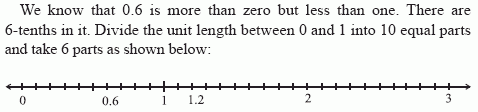Email us to get an instant 20% discount on highly effective K-12 Math & English kwizNET Programs!

Online Quiz (WorksheetABCD)

Questions Per Quiz = 2 4 6 8 10

MEAP Preparation - Grade 5 Mathematics1.72 Decimals on a Number Line

 Read the sequence of decimals given. Find the difference between the second and first decimal. Each succeeding decimal is the difference added to the previous decimal. Example 1: Complete the patters 0.6, 1.2, __Similarly 1.2 is represented. Second decimal - First decimal = 1.2 - 0.6 Difference = 0.6 Hence third decimal = second decimal + difference 1.2 + 0.6 = 1.8 Answer: 1.8 Example 2: Complete the patters 2.3, 2.5, ___, 2.7 Second decimal - First decimal = 2.5 - 2.3 Difference = 0.2 Hence third decimal = second decimal + difference 2.5 + 0.2 = 2.7 Answer: 2.7 Directions: Find the missing decimal in the pattern. Also write at least 10 examples of your own.
 Q 1: Complete the pattern: 4.73, 4.731, 4.732, 4.733, ____, 4.735, 4.736, 4.737, 4.738, 4.739, 4.744.7344.7434.7334.735 Question 2: This question is available to subscribers only!

Subscription to kwizNET Learning System offers the following benefits:

• Unrestricted access to grade appropriate lessons, quizzes, & printable worksheets
• Instant scoring of online quizzes
• Progress tracking and award certificates to keep your student motivated
• Unlimited practice with auto-generated 'WIZ MATH' quizzes